Ex 3.4

Chapter 3 Class 10 Pair of Linear Equations in Two Variables (Term 1)
Serial order wise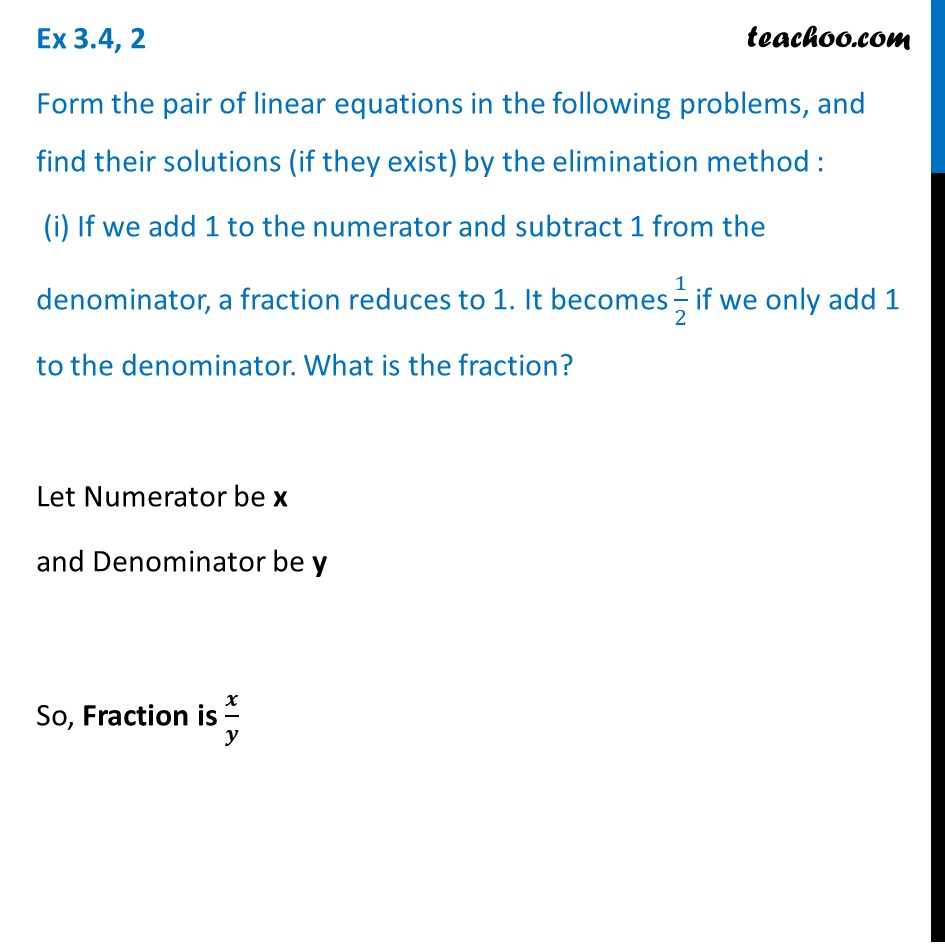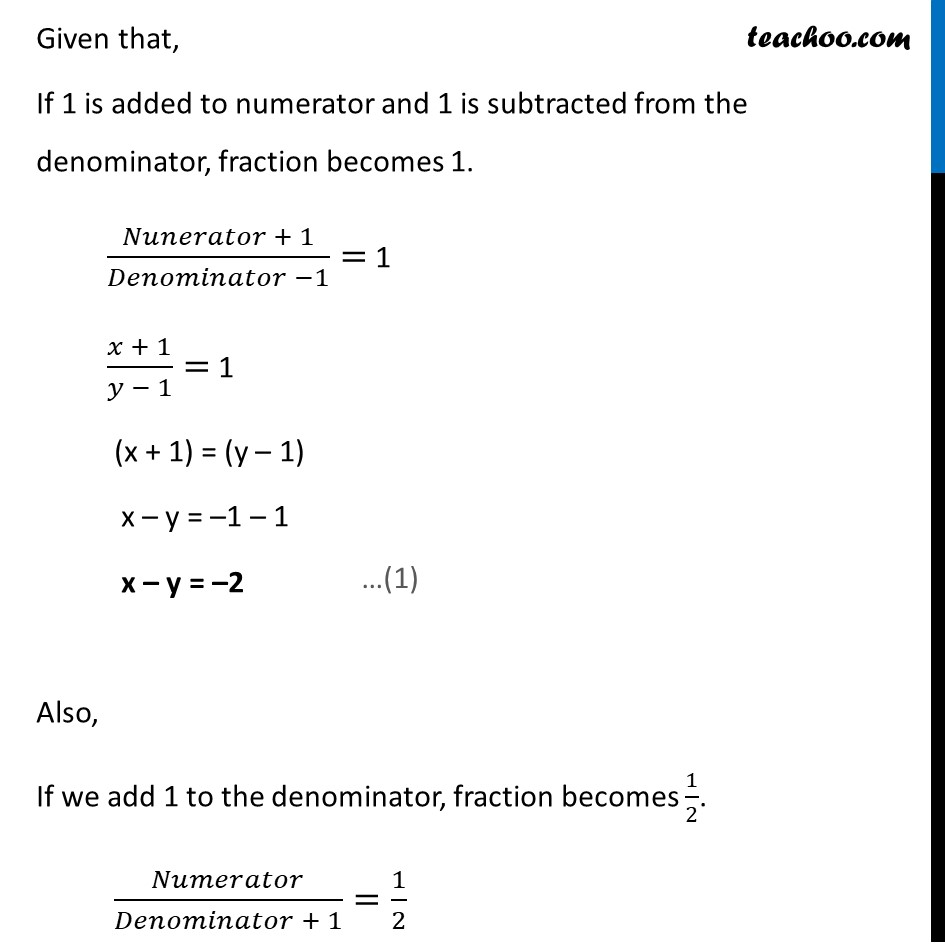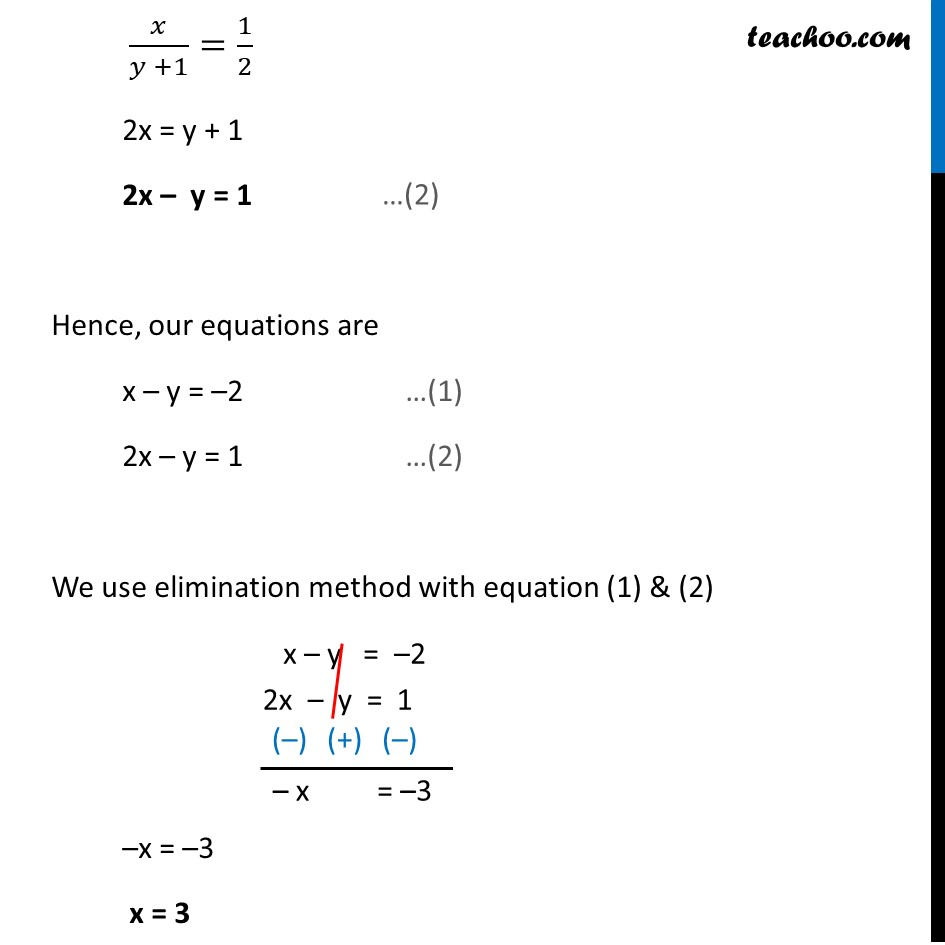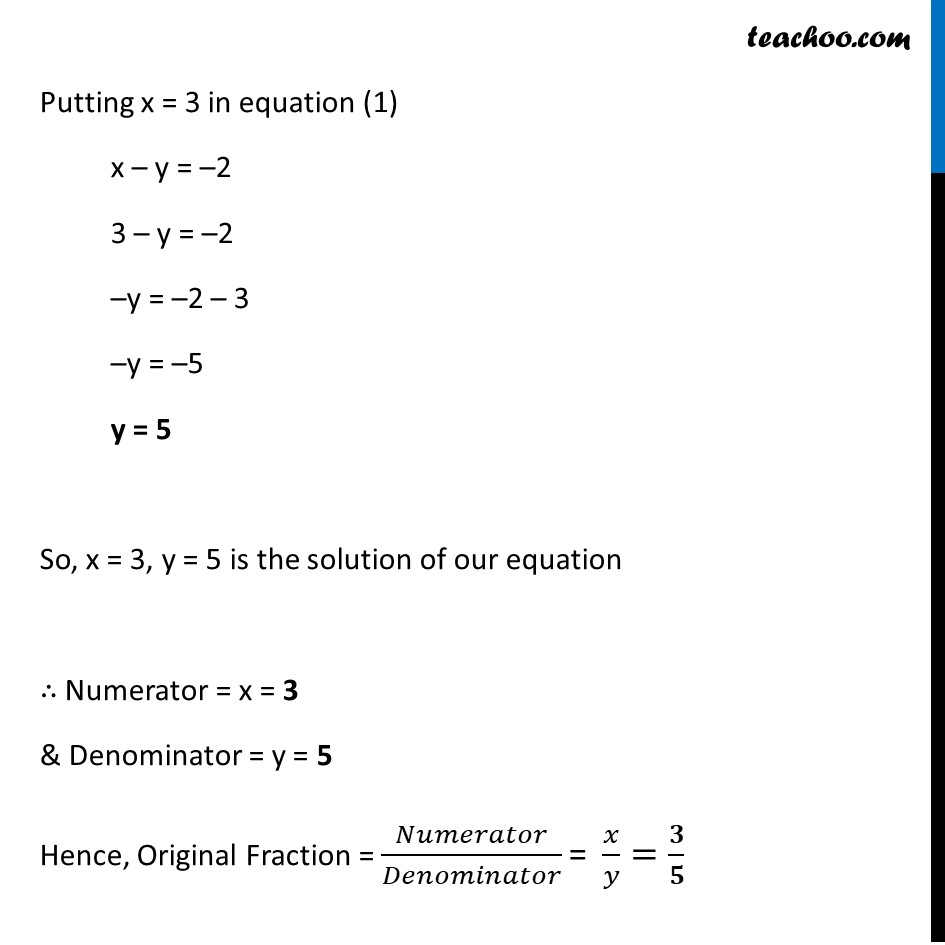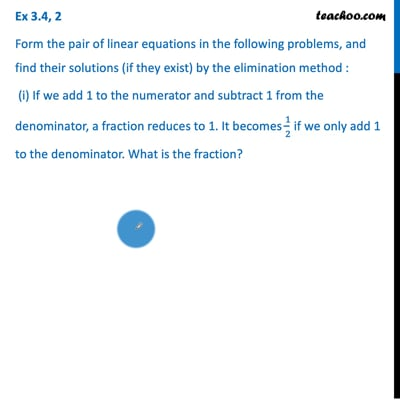This video is only available for Teachoo black users

### Transcript

Ex 3.4, 2 Form the pair of linear equations in the following problems, and find their solutions (if they exist) by the elimination method : (i) If we add 1 to the numerator and subtract 1 from the denominator, a fraction reduces to 1. It becomes 1/2 if we only add 1 to the denominator. What is the fraction? Let Numerator be x and Denominator be y So, Fraction is 𝒙/𝒚 Given that, If 1 is added to numerator and 1 is subtracted from the denominator, fraction becomes 1. (𝑁𝑢𝑛𝑒𝑟𝑎𝑡𝑜𝑟 + 1)/(𝐷𝑒𝑛𝑜𝑚𝑖𝑛𝑎𝑡𝑜𝑟 −1)= 1 (𝑥 + 1)/(𝑦 − 1)= 1 (x + 1) = (y – 1) x – y = –1 – 1 x – y = –2 Also, If we add 1 to the denominator, fraction becomes 1/2. 𝑁𝑢𝑚𝑒𝑟𝑎𝑡𝑜𝑟/(𝐷𝑒𝑛𝑜𝑚𝑖𝑛𝑎𝑡𝑜𝑟 + 1)=1/2 𝑥/(𝑦 +1)=1/2 2x = y + 1 2x – y = 1 Hence, our equations are x – y = –2 …(1) 2x – y = 1 …(2) We use elimination method with equation (1) & (2) –x = –3 x = 3 Putting x = 3 in equation (1) x – y = –2 3 – y = –2 –y = –2 – 3 –y = –5 y = 5 So, x = 3, y = 5 is the solution of our equation ∴ Numerator = x = 3 & Denominator = y = 5 Hence, Original Fraction = 𝑁𝑢𝑚𝑒𝑟𝑎𝑡𝑜𝑟/𝐷𝑒𝑛𝑜𝑚𝑖𝑛𝑎𝑡𝑜𝑟 "= " 𝑥/𝑦=𝟑/𝟓